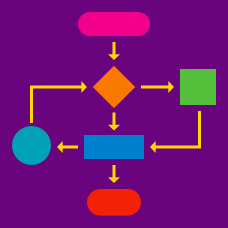Computer Science

# Computer Science Warmups: Level 5 Challenges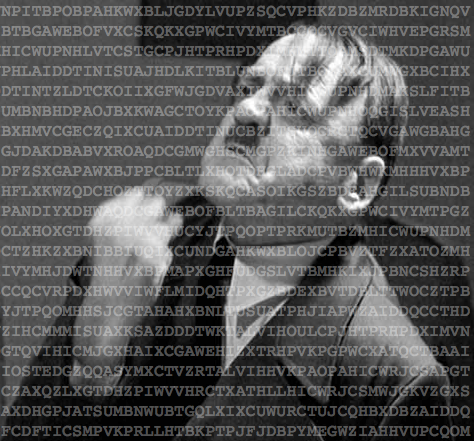It's 1944, and you have just been handed the following cipher text, intercepted from a German command post in France:

There is reason to believe that this text was not encrypted with the sophisticated German Enigma machine but instead used a Vigenère cipher with a simple pass phrase. Thus, it is vulnerable to frequency analysis.

Decode this message and find out where the Germans are sending their bombers so we can evacuate the civilians. You will enter the number of the district (as shown in this list) to submit your answer.

Details and assumptions

A Vigenère cipher is a more complicated shift cipher. For example, rather than shifting every character in a word by two letters like this:

SECRET -> UGETGV


you would instead choose a pass phrase (e.g., "CAT"), which would then tell you to shift each of the letters in your plain text by the letter in the passphrase ("C" is a shift of 2 character, "A" is a shift of none, etc. ) :

C A T C A T (passphrase)
S E C R E T (plain text)

U E V T E M (cipher text)


Note: This problem is loosely based on cryptographic methods used around the time of the invasion of Normandy in 1944.

A toy model for a growing network is the recursive tree. The tree starts as a single node and at each step in the growth process, one new node is added which makes a single connection, at random, to an existing node.

Assume that the network has grown large enough so that its statistical properties are effectively constant. The fractions of nodes that have connections to exactly 7 other nodes is given by some fraction $\dfrac ab$, where $a$ and $b$ are positive coprime integers. What is the value of $a + b$?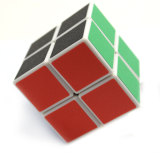Consider the $2\times 2\times 2$ cube puzzle, shown above. It consists of 8 pieces that start out in the solved orientation and can be transformed into alternative orientations by rotating any of the six faces.

To start, let us consider the two moves $\mbox{R}_2$ and $\mbox{U}_2$. When $\mbox{R}_2$ is performed, the right side of the cube is spun two quarter rotations clockwise. Similarly, $\mbox{U}_2$ indicates that the top layer ($\mbox{U}=$ Up) is spun two quarter rotations clockwise. Whenever a face gets two quarter turns, each piece in that face ends up diagonal to its original position on the face.

We start transforming the cube by performing $\mbox{R}_2$ followed by $\mbox{U}_2$. We call this sequence of events the $\mbox{R}_2\mbox{U}_2$ permutation. How many $\mbox{R}_2\mbox{U}_2$ permutations do we go through before the $2\times 2\times 2$ cube is back to its original state?

Note: The $\mbox{R}_2$ and $\mbox{U}_2$ moves are displayed below on a $3\times3\times3$ cube.

• $\mbox{R}_2$• $\mbox{U}_2$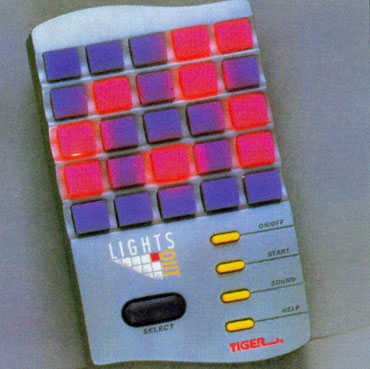Consider an $N \times N$ grid of lights, each one either on or off. The grid can be manipulated by pressing any one light on the grid, toggling the light - however, when a given light is pressed, all lights that are edge-wise adjacent to the pressed light also get toggled.

Given an initial configuration, one might be interested in a sequence of moves that turns all of the lights on the board off, or more simply, whether such a sequence exists or not. For example, the following $5 \times 5$ grid can be solved by pressing the five numbered squares in their labeled order: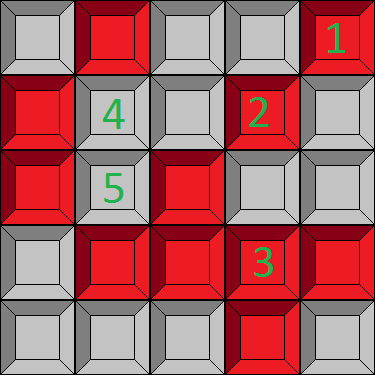As surprising as it may seem, this second board is provably unsolvable. There is no sequence of moves in existence that turns all of the lights off:Grid configurations will be represented as a series of lines in a text file. The first line will be a single integer, $N$, specifying the size of the grid. The following $N$ lines will specify the contents of the grid, where '$\text{\#}$' characters indicate lights that are on, and '$\text{.}$' characters indicate lights that are off. The two grid configurations above would be encoded as:

  1 2 3 4 5 6 7 8 9 10 11 12  5 .#..# #..#. #.#.. .#### ...#. 5 .#... .##.. #.... ..... ..... 

Clicking lights.txt will trigger a download of a ~300KB zip file, which contains a text file named "lights.txt". The text file contains, in the specified format, $10000$ light grids, with sizes $5 \leq N \leq 16$. Precisely $X$ of these grids are solvable.

Find $X$.

Note: An efficient program can find $X$ in well under one minute. If your program is taking hours to complete, you may need to rethink your approach.

###### Image Credit: http://www.edcourtenay.co.uk/musings-of-an-idiot/2002/04/10/LightsOut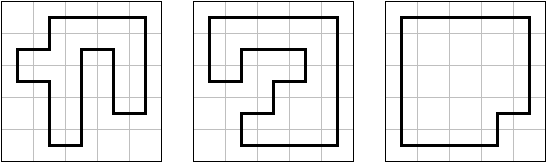In the world of pencil puzzles, there are many puzzle types where you have to draw a loop on a lattice grid, including Country Road, Masyu, Pure Loop, Slalom, and Yajilin. In most of these puzzles (and all of the linked above), the loop visits some of the cells, passing through the cells' centers, and may not use a cell more than once (which also means no intersections, no touching itself, etc).

Formally, on a polyomino $P$, a loop is a sequence of $n \ge 4$ squares $(a_1, a_2, a_3, \ldots, a_n)$ such that all squares $a_i$ are in $P$, $a_i$ and $a_{i+1}$ share a side for all valid $i$, $a_n$ and $a_1$ also share a side, and all squares in the loop are distinct. Loops are cyclic (it can start from any square in the loop) and don't have any orientation (reversing the loop doesn't matter), thus $(a_1, a_2, a_3, a_4), (a_2, a_3, a_4, a_1), (a_4, a_3, a_2, a_1)$ all describe the same loop.

Determine the number of loops on a $5 \times 5$ square.

×